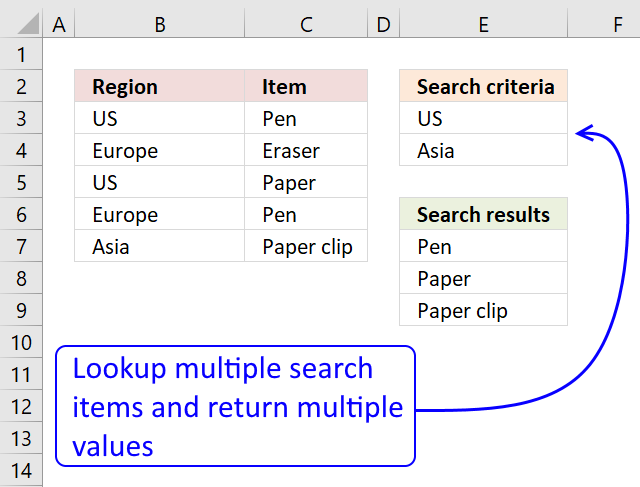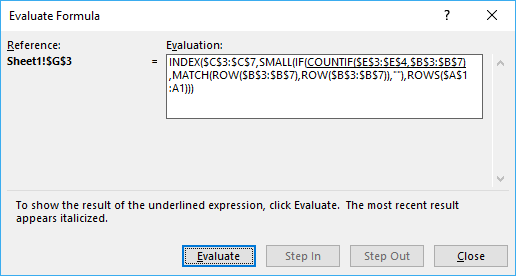Author: Oscar Cronquist Article last updated on February 14, 2018

In this article I´ll show you how to lookup two or more values in a list and return (if possible) multiple matches.

The picture below shows you a table in column B and C, the search criteria is in column B and the results are in column G.I am not using VLOOKUP at all in this array formula, the VLOOKUP looks for a value in the leftmost column of a table, and then returns a value in the same row from a column you specify.

The VLOOKUP function is not designed to look for multiple values and return multiple values.

Array formula in E7:

=INDEX(\$C\$3:\$C\$7, SMALL(IF(COUNTIF(\$E\$3:\$E\$4, \$B\$3:\$B\$7), MATCH(ROW(\$B\$3:\$B\$7), ROW(\$B\$3:\$B\$7)), ""), ROWS(\$A\$1:A1)))

Recommended article

Smallest greater than condition

Question: Here is the problem: i have a data table with 2 columns: A B 2.93 12.8 2.94 12.2 3 […]

### How to enter an array formula

1. Copy the aray formula above (Ctrl + c)
2. Double click cell G3
3. Paste (Ctrl + v)
4. Press and hold Ctrl + Shift simultaneously
5. Press Enter
6. Release all keys

If you made the above steps correctly the formula now has a beginning and ending curly bracket, like this:
{=array_formula)}

Don't enter these characters yourself, they appear automatically.

How to enter an array formula

Array formulas allows you to do advanced calculations not possible with regular formulas.

### How to copy formula to cells below

1. Select cell E7
2. Copy cell (Ctrl + c)
3. Select cell range E8:E9
4. Paste (Ctrl + v)

### How the array formula works in cell E7

You can easily follow a long as I explain the array formula, download the workbook. Select cell B13, go to tab "Formulas". Click on "Evaluate formula" button.Click on "Evaluate" button show above to move to next step.

#### Step 1 - Count matching search criteria in column B

COUNTIF(\$E\$3:\$E\$4, \$B\$3:\$B\$7)

becomes

COUNTIF({"US";"Asia"},{"US";"Europe";"US";"Europe";"Asia"})

and returns {1;0;1;0;1}

How to use the COUNTIF function

Counts the number of cells that meet a specific condition.

#### Step 2 - Convert boolean array into corresponding row numbers

IF(COUNTIF(\$E\$3:\$E\$4, \$B\$3:\$B\$7), MATCH(ROW(\$B\$3:\$B\$7), ROW(\$B\$3:\$B\$7)), "")

becomes

IF({1;0;1;0;1}, MATCH(ROW(\$B\$3:\$B\$7), ROW(\$B\$3:\$B\$7)), "")

becomes

IF({1;0;1;0;1}, {1;2;3;4;5}, "")

and returns {1;"";3;"";5}

These are the row numbers that correspond to the matching values US and Asia in column B.

How to use the IF function

Checks if a logical expression is met. Returns a specific value if TRUE and another specific value if FALSE.

#### Step 3 - Returns the k-th smallest row number

SMALL(IF(COUNTIF(\$E\$3:\$E\$4,\$B\$3:\$B\$7), MATCH(ROW(\$B\$3:\$B\$7), ROW(\$B\$3:\$B\$7)), ""),ROWS(\$A\$1:A1))

becomes

SMALL({1;"";3;"";5}, ROWS(\$A\$1:A1))

becomes

SMALL({1;"";3;"";5}, 1)

and returns 1.

How to use the SMALL function

The SMALL function lets you extract a number in a cell range based on how small it is compared to the other numbers in the group.

#### Step 4 - Return a value based on coordinate

INDEX(\$C\$3:\$C\$7, SMALL(IF(COUNTIF(\$E\$3:\$E\$4, \$B\$3:\$B\$7), MATCH(ROW(\$B\$3:\$B\$7), ROW(\$B\$3:\$B\$7)), ""), ROWS(\$A\$1:A1)))

becomes

INDEX(\$C\$3:\$C\$7, 1)

becomes

INDEX({"Pen";"Eraser";"Paper";"Pen";"Paper clip"}, 1)

and returns Pen in cell E7.

How to use the INDEX function

Gets a value in a specific cell range based on a row and column number.

### Download excel *.xlsx file

Vlookup with multiple search conditions and return multiple matches.xlsx